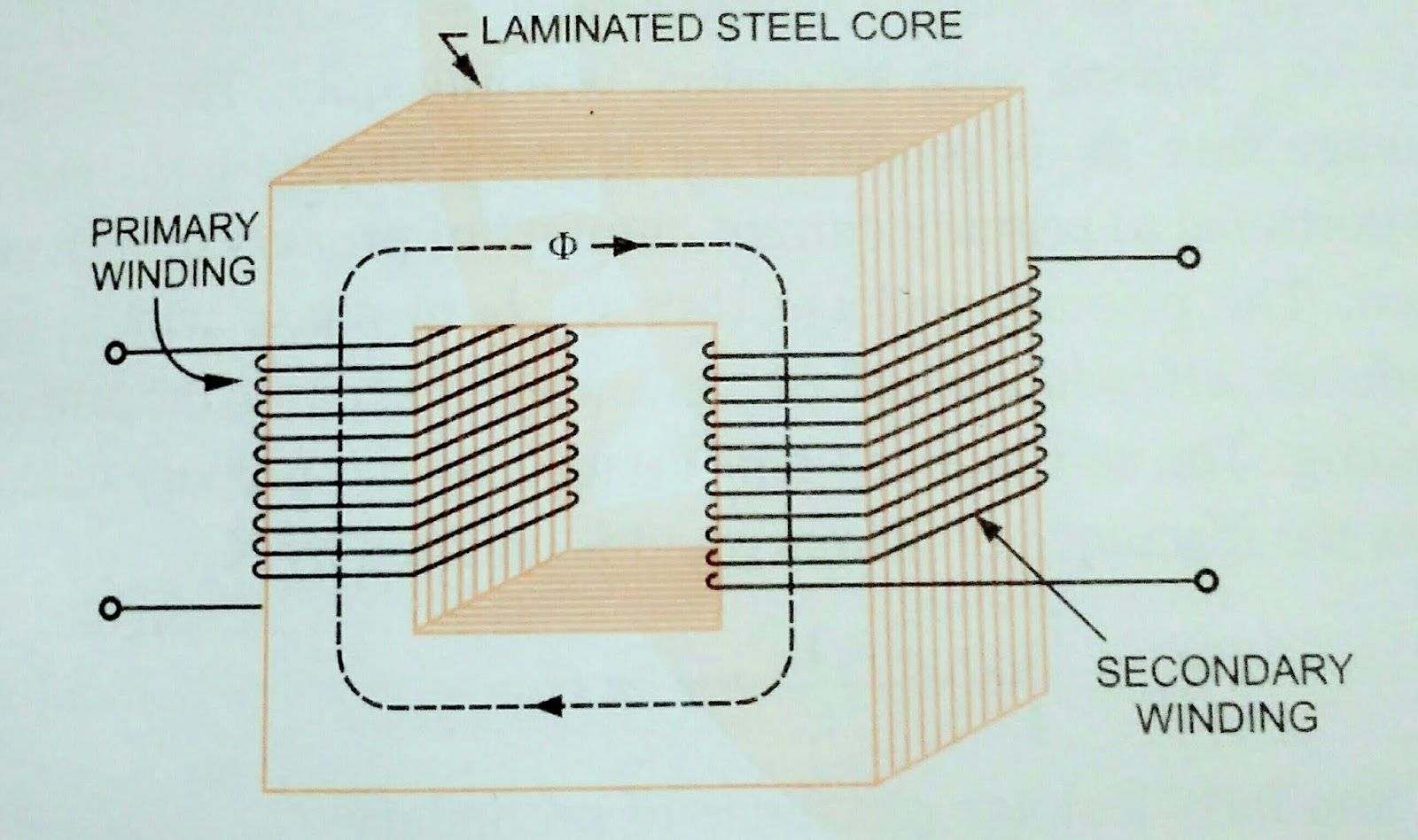### Ticker

6/recent/ticker-posts

# MACHINE [ TRANSFORMER ] CHAPTER :- 1

How to Learn about of BASIC INFORMATION OF TRANSFORMERS ???????

Transformer is an AC Machine that
I] Transfer Electrical Energy from one Electric Circuit to another

II] does so without a change of frequency

III] does so by the Principle of Electromagnetic induction and

IV] has Electric Circuit that are linked by a common magnetic Circuit.

The energy transfer usually takes place with a change of voltage, although to this is not always necessary. It is a static Machine and has very high efficiency ( in the range of 96% to 99%).
A transformer is never operated on DC. If dc supply is connected to the transformer primary to secondary , it may burn out since there is self induced  EMF and heavy Current will flow through it.
Although in the actual construction the two windings are usually wound one over the other, for the sake of simplicity,the figure for analyzing transformer theory show the windings on opposite sides of the core, as shown in figure.Simple Transformer
Simple Transformer

Ideal Transformer.

In an Ideal transformer, windings resistance, magnetic leakage, and iron losses are neglected and core is assumed to have infinite permeability and zero reluctance.

EMF Equation.

The EMF induce in primary and secondary windings of a transformer are given as follows

E1 = 4.44 f×N1 Φ max volts ----- (1)
E2 = 4.44 f×N2 Φ max volts -----(2)

Where Φ max is the Maximum value of Flux is werbes, f is the supply frequency in
Hz , N1 is the number of tuns on primary windings and N2 is the number of tuns secondary windings.
In the Ideal transformer, the voltage drops in primary and secondary windings are negligible and therefore E1 Will be approximately equal and opposite the voltage impressed across primary, V1 and terminal Voltage V2 will be approximately equal to E2.
So voltage ratio,

V2       E2       4.44 f N2 Φmax       N2
---- = ---- = ------------------- = ----- -->(3)
V1       E1       4.44 f N1 Φmax       N1

Voltage Transformation Ratio

The ratio of secondary Voltage to primary voltage is Known as the voltage Transformation  ratio and is designated by letter K.
i.e., Voltage Transformation ratio,

V2        E2         N2
K = ------ = ------ = ------ -----(4)
V1        E1         N1

Current Ratio

The ratio of secondary Current to primary current is known as current ratio and reciprocal of voltage Transformation ratio is an ideal Transformer.

I2       V1      E1      N1      1
i.e.,    ----- = ---- = ---- = ---- = -----
I1        V1     E2       N2     K

Effects of Voltage and Frequency Variations.

If the transformer is operated with the frequency and voltage changed in the same Proportion, the flux density will remain unchanged as obvious from Eq.
(2)  and apparently the no load Current will also remain unaffected.
The transformer can be operated safely at frequency less than rated one with corresponding reduce voltage. In this case iron losses will be reduced. But if the transformer is operated with increased Voltage and Frequency in the same Proportion, the core may increase to an intolerable level. Increase in frequency with constant supply voltage will cause reduction in Hysteresis loss and leave the Eddy Current losses unaffected.
Some increase in voltage could, therefore, be tolerated at higher frequencies, but exactly how much depends on the relative magnitude of the Hysteresis and Eddy Current losses and the grade of iron used in the transformer core.